# Learning to drive (from a self-driving car!)

Humans are the most complex organism known to the whole history of the universe. Our ability to make rational decisions and process millions of possible outcomes within a matter of seconds has allowed us to dominate the world, procreate, and advance technological evolution.

Although often overlooked, driving is one of the most remarkable actions a human can perform. The ability to process several different outcomes while being able to understand the location you’re at, understand the traffic signs and lights in front of you, and being able to predict the path other drivers will follow is simply exceptional.

But can you code the same, rational thinking process into an autonomous vehicle? How does a self-driving car think like a human? How does it make decisions with regard to the direction it should continue to drive in? Let’s take a look at Path Planning, an integral field of the development of autonomous vehicles.

# Note: Frenet Coordinate System

The article will be covering the use of Frenet coordinates, an alternate coordinate system that can be used to help map out the car’s actions on a road in more of an intuitive way than the traditional x y coordinates.

This is how our car would look like when displayed in the Cartesian coordinate system: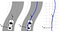Source

This is how we can represent a car using the Frenet Coordinate system.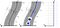Source

As depicted from the second image, the frenet coordinate system allows for a proper system where the road can be successfully mapped and we would not have to deal with the recursive problem of mapping the road at every single timestamp as a polynomial.

The s coordinate is defined to be the length of the road and can be depicted as the longitudinal positions of the car with respect to its reference path. d is the lateral direction of the car with respect to the center of the lane. The center of the lane, as depicted in blue, can be thought of as the “y” axis where it takes the shape of the road.

We can now define our variables, s and d, and their accelerated positions, velocities, and accelerations. Because we initially don’t define the relationship between s and d, we can assume that both variables are independent and initialize their positions, velocities, and accelerations.

# The motion planning problem

We can define the motion planning problem as given the start configuration (qstart → this will be given from localization and sensors), a goal configuration (qgoal), and the constraints in the environment (physics, map, traffic, etc.), find a sequence of moves in the configuration space (all possible configurations of the car → can be in [x, y, θ] where θ is our heading) that can move the robot from qstart to qgoal without colliding with any obstacles.

The overarching path planning process can be broken down into 3main subsystems: prediction, behaviour planning, and path planning.

# Predicting the actions of other cars

We can first start off the overall problem through predicting the actions of other cars given a certain timespan. We want to be able to predict and deduce the actions of other vehicles so that the self-driving car is able to drive without colliding into other vehicles while optimizing for the best path required.

The way we can handle multi-model uncertainty beliefs of an object is through a method that we can use to help keep track of our prior beliefs through a Gaussian distribution.

This will allow us to model the car in such a way that we can successfully make a prediction as to where the car will go.

In the prediction pipeline, the 2 main processes we can generally take is a model-based approach and a data-driven approach.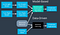Example pipeline of a model-based vs data-driven approach. Source

The model-based approach allows us to incorporate the knowledge we have about physics, constraints that are imposed by the road, etc. making this a computationally efficient algorithm.

On the other hand, the data-driven approach allows us to recognize patterns that might not have been recognized via a black-box Machine Learning algorithm pipeline. An example of a data-driven approach can be Trajectory Clustering.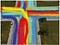Source

By taking in input data of past polynomial trajectories taken by other vehicles, the unsupervised ML algorithm will allow us to characterize and define x amount of base paths (like an average) of the paths taken for every action (left, right, straight). We would then simply fit the action of a self-driving car against a probability distribution to figure out the likelihood of a certain direction. Here’s the paper for more information.

What we can do is combine an ML classifier with a filter. This can be done using a Gaussian Naive Bayes algorithm to help successfully complete this task. Because of the fact that we can study and learn from input trajectory data while being able to select the key features that matter to us, the Gaussian Naive Bayes algorithm will allow the model to use probability distributions to help account for uncertainty while making proper predictions.

Given a new data point, the prediction model requires 2 steps:

1. Compute the conditional probabilities for the given [x, y] pairings. This can be modelled as

where x is the feature, C is the label, with mean μ, and standard deviation σ which is computing during the training process.

2. Use the traditional conditional probability in a Naive Bayes classifier to take the hypothesis which is the most profitable.

# Behaviour Planning

The behaviour planning problem can be summarized as given the input of the map, the route that we will be taking from start to end, and the predictions that we’ve acquired of the other objects, create a path that can bring the car to the destination, collision-free, smooth, and safe.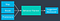Source

We need to be able to suggest states that are:

1. Feasible
2. Safe
3. Legal
4. Efficient

The approach we can use to solve this problem is through the use of a Finite State Machine (FSM). We can essentially create a pipeline where we can make decisions based on the finite set of discrete states that we can take. Building an FSM will allow us to make logical and sequential states for high-level situations that the car might face when driving.

For example, if a car in front of us is driving at an extremely slow speed, then we might create a transition function that can bring us into either the left lane or the lane to the right of us. We might have another node branching out from this condition telling the pipeline that if there is a car in either the left lane or the right lane, then we need to go to the right lane.

The video linked below further explains the use of FSMs and how they work through the use of visuals:

# Polynomial Trajectory Generation

Now that we’ve built a model that will plan for typical situations that might occur when driving, we now need to plan for a specific path that we can use in order to successfully move the car and adjust its speed.

One thing to note is that when we build a suitable trajectory, it needs to be in respect to time; therefore, we’d have a 3-dimensional vector consisting of [s, d, t] where t is the time. Our path has to be executed while taking into account where the other cars will be at a certain time step (thus, driving in traffic is a 3-dimensional problem).

In order to produce a successful trajectory, there are some requirements it must follow in order to create a successful path. The main condition we have to keep in mind is jerk. Having a higher jer has been shown to be uncomfortable. Focusing on this specific aspect can allow us to create a trajectory model while limiting the amount of jerk.

We define the total squared jerk to be

where the jerk value itself is defined to be as s(t)² (note that the s means the 3 dots in the equation → medium doesn’t support latex embedding) which can be otherwise known as the third derivative of our position with respect to time. The goal of the total squared jerk function is to be minimized by creating a function, s(t).

If you chose to go through the math behind the total squared jerk, you’ll realize that all the time derivatives of s after the 6th order will have to be 0 in order for s to be jerk minimal.

Using that realization, we can define s(t) with 6 coefficients:

Note: this also applies to the lateral displacement, d(t).

Using the equations provided above, we can take the first and second-order derivatives in order to successfully get the equations for the velocity and acceleration equations respectively:Velocity equation (first-order derivative)Acceleration equation (second-order derivative)

But what happens when we set the initial time as 0? When we do that, we find that,

Because of this, we can now simply our equations to become

If we put this all in matrix form by separating the coefficients from the unknown variables (α), we get,

The way we can grab the coefficients for α_3, α_4, and α_5 is by taking the inverse of the time coefficients and multiplying that be the output vector.

Isn’t that simply amazing!? By taking the inverse of the matrix on the left and multiplying that by the output vector, we’re not only able to get the 3 missing variables but we can now fit them into the original equation

and get an output polynomial that we can use as a viable path!

# Results

I’ve implemented this in C++ and here you’ll be able to find the outcome of the Jerk Minimization Trajectory implemented in Udacity’s simulator below:

Feel free to take a look at my Github:

# Connect with me

Email

Linkedin

Twitter

Website

Want to hear more? Subscribe to my newsletter.

Building Self-Driving Cars as a 14yo. srianumakonda.com

Building Self-Driving Cars as a 14yo. srianumakonda.com